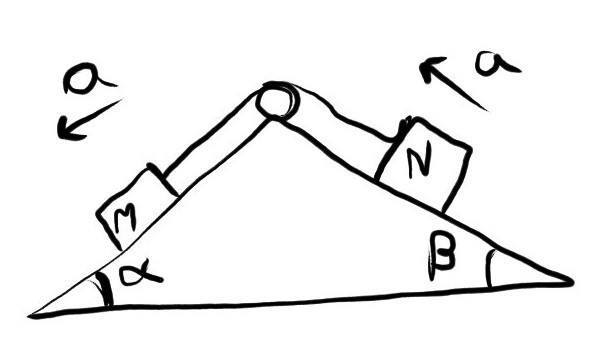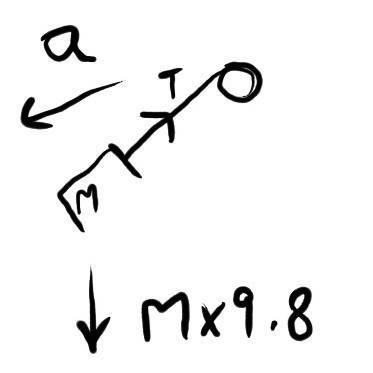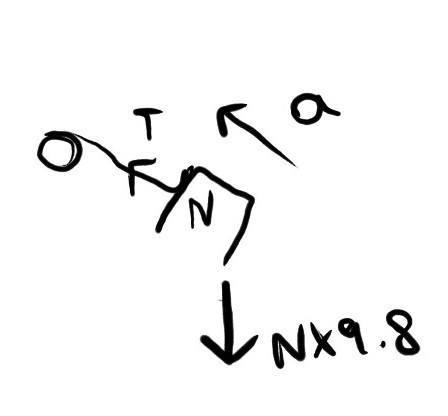# Newtons Law Of Motion Problem

To Admin - This is not my homework, My teacher solved it but I am trying to solve this problem using other methods. If I posted this in wrong group, please message me and I will Post this in correct corner.
Problem - To find acceleration in both blocks sliding on smooth surface,string and pulley is frictionless and massless. Refer to diagram below.Use g = 9.8 m/s2My Solution -
Free Body Diagram Of Block of mass M kg -The Equation of forces in Y axis is
9.8M - Tsinα = Macosα .......eq(1)
The Equation of forces in X axis is
Tcosα = MAsinα ...........eq(2)

Free Body Diagra of Block of Mass N kg -The Equation of forces in Y axis is
Tsinβ - 9.8N = Nasinβ .........eq(3)
The Equation of forces in X axis is
Tcosβ = Na.cosβ ..........eq(4)

Now, after simultaneously solving eq(1) and eq(3), I am getting wrong answer
Now, eq(4) is tell that T = Na which is not possible.
I guess I missed Normal Reaction Force but how to use it in equation

#### Attachments

Doc Al
Mentor
Yes, you definitely missed the Normal force. Add it to your diagram and equations like any other force.

Life would be much easier if you chose coordinates parallel to the planes rather than vertical and horizontal.

The Equation of forces in Y axis is
9.8M - Tsinα = Macosα .......eq(1)
The Equation of forces in X axis is
Tcosα = MAsinα ...........eq(2)

You have resolved the forces incorrectly.The normal force from the wedge will have a component in both x and y direction.